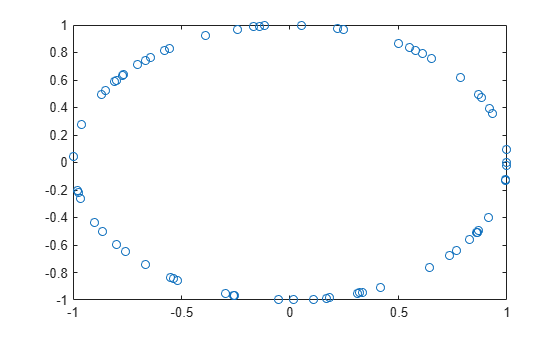Main Content

# ltePSBCHDRS

PSBCH demodulation reference signal

## Syntax

``````[seq,info] = ltePSBCHDRS(ue)``````

## Description

example

``````[seq,info] = ltePSBCHDRS(ue)``` returns a 144-by-1 complex column vector sequence containing PSBCH demodulation reference signal (DM-RS) values and an associated information structure for the specified UE settings structure. For more information, see PSBCH Demodulation Reference Signal.```

## Examples

collapse all

Generate a PSBCH DM-RS sequence associated with both DM-RS SC-FDMA symbols in a subframe.

```ue.NSLID = 170; [psbchdrs_seq,info] = ltePSBCHDRS(ue);```

Plot the DM-RS sequence (real vs. imaginary).

`plot(psbchdrs_seq,'o')`## Input Arguments

collapse all

User equipment settings, specified as a parameter structure containing this field:

Sidelink mode, specified as `'D2D'` or `'V2X'`.

Data Types: `char` | `string`

Physical layer sidelink synchronization identity, specified as an integer from 0 to 355. (${N}_{\text{ID}}^{\text{SL}}$)

Data Types: `double`

Data Types: `struct`

## Output Arguments

collapse all

PSBCH DM-RS values, returned as a column vector. For the D2D sidelink mode, `seq` is a 144-length column containing the values for each DM-RS symbol in each slot in a subframe. For V2X, it is a 216-by-1 complex column containing the concatenated values for the three DM- RS symbols in a subframe.

Data Types: `double`
Complex Number Support: Yes

PSBCH DM-RS information about the intermediate variables used to create the DM-RS, returned as a parameter structure containing these fields:

Reference signal cyclic shift for each slot, returned as a two-column vector. (α)

`Alpha` is proportional to `NCS`, where $\alpha =\frac{2\pi {n}_{cs,\lambda }}{12}$.

Base sequence group number for each slot, returned as a two-column vector. (u)

Base sequence number for each slot, returned as a two-column vector. (v)

Root Zadoff-Chu sequence index for each slot, returned as a two-column vector. (q)

Cyclic shift values for each slot, returned as a two-column vector. (${n}_{\text{cs,}\lambda }$)

Zadoff-Chu sequence length, returned as an integer. (${N}_{ZC}^{RS}$)

Orthogonal cover value for each slot, returned as a matrix. ($\overline{w}$)

Data Types: `struct`

## More About

collapse all

### PSBCH Demodulation Reference Signal

The PSBCH demodulation reference signal (DM-RS) is transmitted alongside the `ltePSBCH` values in the central 72 resource elements and in two SC-FDMA symbols in a synchronization subframe for D2D sidelink mode and three SC-FDMA symbols for V2X.. For zero-based indexing, the SC-FDMA symbol indices are {3,10} for normal cyclic prefix and {2,8} for extended cyclic prefix. These are the same symbols used by the PUSCH DM-RS, see `ltePUSCHDRSIndices`. For the V2X sidelink mode, the symbol indices are {4,6,9}.

Note

The indicated symbol indices are based on TS 36.211, Section 9.8, but expanded from symbol index per slot to symbol index per subframe to align with the LTE Toolbox™ subframe orientation. For more information on mapping symbols to the resource element grid, see Resource Grid Indexing.

### PSBCH Demodulation Reference Signal Indexing

Use the indexing function, `ltePSBCHDRSIndices`, and the corresponding sequence function, `ltePSBCHDRS`, to index the resource grid for any synchronization subframe number. The indices are ordered as the PSBCH DM-RS symbols should be, applying frequency-first mapping, into the two DM-RS SC-FDMA symbols. For more information on mapping symbols to the resource element grid, see Resource Grid Indexing.

 3GPP TS 36.211. “Evolved Universal Terrestrial Radio Access (E-UTRA); Physical Channels and Modulation.” 3rd Generation Partnership Project; Technical Specification Group Radio Access Network. URL: https://www.3gpp.org.